# Lesson Notes By Weeks and Term - Primary 2

TERM: 1st Term

WEEK: 7

CLASS: Primary 2

AGE: 7 years

DURATION: 5 periods of 40 minutes each

DATE:

SUBJECT: Mathematics

SPECIFIC OBJECTIVES: At the end of the lesson, the pupils should be able to

1. Subtract 2-digit numbers using the column method.
2. Practice subtracting 2-digit numbers using the column method.
3. Solve 2-digit subtraction problems using a number line.
4. Solve addition problems using bar diagrams.

INSTRUCTIONAL TECHNIQUES: Explanation, question and answer, demonstration, practical

INSTRUCTIONAL MATERIALS: Printed tens, bottle tops and place value table per learner,

PERIOD 1: Column subtraction

PRESENTATION

TEACHER’S ACTIVITY

PUPIL’S ACTIVITY

STEP 1

MENTAL MATHS

The teacher begins the lesson with some mental calculations

Subtract the following

1 9 – 2 =

2 6 – 4 =

3 7 – 3 =

4 8 – 6 =

5 10 – 2 =

6 12 – 1 =

7 15 – 3 =

8 17 – 5 =

9 19 – 3 =

10 20 – 5 =

Pupils respond and participate

STEP 2

CONCEPT

DEVELOPMENT

The teacher

Writes the following number sentence on the board 39 – 14 = ______

Asks: How many tens and ones in 39? (3 tens and 9 ones). Put the tens and ones into the place value table to prepare to subtract.

Asks: How many tens and ones in 14? (1 ten and 4 ones). This is what we must take away from 39.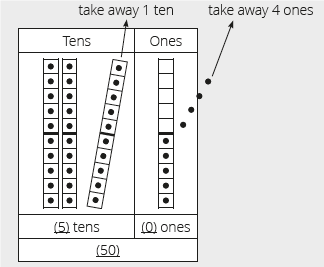Asks: How can we solve this subtraction problem using the place value table?

Lets the learners share their ideas.

You will need to guide them in this discussion. Ensure that they make use of the terms tens, one, subtract.

Use the diagram above to help you explain how you subtract the tens and ones.

In the tens place I take away 1 ten from 3 tens. I am left with 2 tens.

In the ones place I take away 4 ones from 9 ones. I am left with 5 ones.

CLASS ACTIVITY

The teacher

Writes 39 – 14 = ____ on the board.

Explains to the learners that we will now be solving this question using the

column method.

Lets learners think and discuss how they can write the subtraction in columns.

Lets some learners present their ideas on the board. If nobody can show the correct column method, draw the diagram below and talk through the steps

 T O 3 9 - 1 4 5 2 0 2 5

O: 9-4 = 5

T: 30 – 10 = 20

You must discuss each step. Ensure that you use the correct language- ones, tens, subtract.

Even if learners presented the correct column method, you have to repeat the steps to go over them with the whole class.

• The first step is to line up the numbers vertically in tens and ones.

• Subtracts the ones column: 9 – 4 = 5 (use bottle tops to show this subtraction)

• Subtracts the tens column: 30 – 10 = 20 (use printed tens to show this subtraction)

• Now adds the tens and ones together: 20 + 5 = 25 (how many ones and how many printed tens do you have left?)

Writes the following on the board: 28 – 16 =

Repeats the above steps with the learners for this second example.

ACTIVITY II

The teacher

Writes the following number sentence on the board: 39 – 14 = ___

Writes: 39 – 14 = 30 + 9 – 10 – 4

= 30 – 10 + 9 – 4

= 20 + 5

= 25

Explains to the learners that we write the tens next to each other and then subtract them from each other (30 – 10 = 20) and the ones (9 – 4 = 5)

Then we add the tens and ones together. The answer is 25.

Pupils pay attention and participate

STEP 3

CLASS-WORK

Calculate the following using column method

1. 35 - 12=

2. 88 – 16 =

3. 69 – 28 =

4. 76 – 33 =

5. 98 – 72 =

Pupils attempt their class work

STEP 4

HOME-WORK

a 77 – 65 =

b 86 – 51 =

c.67 - 13 =

The pupils writes it in their homework book

STEP 5

SUMMARY

The teacher summarizes by reminding the pupils that in this lesson we solved subtraction problems. Firstly we used the place value table and then moved onto learning the column method. We will be practising this method for the next few lessons.

She marks their class works, makes corrections where necessary and commends them positively

PERIOD 2: Column Subtraction

PERIOD 3: Subtraction using a number line

PRESENTATION

TEACHER’S ACTIVITY

PUPIL’S ACTIVITY

STEP 1

MENTAL MATHS

The teacher begins the lesson with some mental calculations

Which numbers are between

1. 21 and 18

2 23 and 20

3 19 and 22

4 2 and 6

5 8 and 11

6 13 and 17

7 15 and 19

8 18 and 16

9 15 and 12

10 10 and 6

Pupils respond and participate

STEP 2

CONCEPT

DEVELOPMENT

The teacher

• Writes 25 – 13 = ___ on the board.

• Ask the learners if they remember the method that they learnt yesterday. (The column method.)

• Revises the steps with the learners solving 25 – 13 =

• The first step is to line up the numbers vertically in tens and ones.

 T O 2 5 - 1 3 2 1 0 1 5

O: 5 -3 = 2

T: 20 – 10 = 10

Subtracts in the ones column: 5 – 3 = 2 (show using bottle tops if necessary)

Subtracts in the tens column: 20 – 10 = 10 (show using printed tens if necessary)

Now adds the tens and ones together: 10 + 2 = 12

CLASS ACTIVITY

The teacher

In this activity we will be solving the same question 25 – 13 = ____ using a number line.

Draws a number line on the board – label 25 on the right hand side of the number line.

Asks: Why do we label 25 on the right hand side of the number line? (we want to subtract from 25.)

Ask: How many tens and ones in 13? (1 ten and 3 ones)

Explains to the learners that we will move along the number line to show subtracting 10 first, then 3, from 25.

Draws a single jump of 10 to land on 15. Label 15 on the number line..

Draws a smaller jump of 3 to land at 12. Label this on the number line. The sketch on the board will now look like this: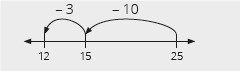Asks: What is difference between the column method and the number line? Which method/way of subtraction did they find easier? Why? (Answers will vary. Listen

respectfully to learners’ answers and discuss the differences between the methods.

Encourages learners to look for the method they find easiest and best to do the calculations.)

ACTIVITY II

The teacher

• If time allows solve the following question with your learners 88 – 16 = ____ (72) using both the column method and number line.

• Activity 1 and 2 can guide you through the steps for each method.

• Remembers to compare the two methods with the learners..

Pupils pay attention and participate

STEP 3

CLASS-WORK

Note: Learners should still use their base ten kits when they do these calculations if necessary.

Calculate the following using the column method and a number line:

a 38 – 27 =

b. 67 – 13 =

c. 76 – 33 =

Pupils attempt their class work

STEP 4

HOME-WORK

Calculate the following using the column method and a number line:

a. 98 -72 =

b. 77 – 65 =

The pupils writes it in their homework book

STEP 5

SUMMARY

The teacher summarizes by reminding the pupils that in today’s lesson we solved subtraction problems. We solved them using both the place value

table and number lines. We also compared the methods and discussed which we found easier and why.

She marks their class works, makes corrections where necessary and commends them positively

PERIOD 4: Addition using bar diagrams

 PRESENTATION TEACHER’S ACTIVITY PUPIL’S ACTIVITY STEP 1MENTAL MATHS The teacher begins the lesson with some mental calculationsWhat is the answer1 6 + 5     2 5 + 8     3 9 + 6     4 8 + 9     5 5 + 7    6 12 + 57 14 + 28 5 + 129 2 + 1110 7 + 13 Pupils respond and participate STEP 2CONCEPTDEVELOPMENT The teacher• Writes the following word problem on the board. This is an example of a‘combine’ question.• Thelma has 13 blue marbles and 4 red marbles. How many marbles does Thelma have altogether?• The first step is to understand the question properly.• In the step 1, we write the word problem on the board. Next, we read the problem.Then, we let the learners read the problem themselves to deepen their understanding.After that we underline the numbers. These are 13 and 4.• First, uses bottle tops to show 13 and 4. Lets the learners count out the bottle tops and add them together on their own.• Then, draws circles to represent the situation.• Finally, draws the following diagram on the board:• Lets learners copy the diagram into their classwork books, then write the number sentence on the board: 13 + 4 = __• Repeats the problem with the bottle tops with the learners. Show them that the bottle tops are represented by the bar diagrams on the board.• Discusses the answer with the learners. (17 marbles). Thelma has 13 blue marbles and 4red marbles. How many marbles does Thelma have altogether? 13 + 4 = 17. He has 17marbles altogether.• This is an example of an addition problem using combine.• Learners record the full number sentence into their classwork books.• Do not erase this diagram from the board. CLASS ACTIVITYThe teacher• This second activity uses addition of compare.• Write the following word problem on the board.• Thelma has 12 sweets. Ben has 5 more sweets than Thelma. How many sweets does Ben have? • The first step is to understand the question properly.• In the step 1, we write the word problem on the board. Next, we read the problem.Then, we let the learners read the problem themselves in case they have any questionsabout what it means.• After that we underline the numbers. These are 12 and 5.• First, use bottle tops to show 12 and 5. Let the learners count out the bottle tops and addthem together on their own.• Next, draw circles to represent the situation.• Finally, draw the following diagram on the board:• Lets learners copy the diagram into their classwork books, then write the number sentence on the board: 12 + 5 = __• Repeats the problem with the bottle tops with the learners. Show them that the bottle tops are represented by the bar diagrams on the board.• Discusses the answer with the learners. (17 marbles). Thelma has 12 sweets. Ben has 5more sweets then Thelma. How many sweets does Ben have? 12 + 5 = 17. Ben has17 sweets.• Learners record the full number sentence into their classwork books.• This is an example of an addition problem of compare.• Do not erase this diagram from the board.• Look at the difference between the combine and the compare diagrams. Both lead to addition. But the amounts are shown in different ways. Pupils pay attention and participate STEP 3CLASS-WORK (This classwork should be done as a class so that yourself and the learners can discuss and solve the problems together.) Write the question and draw a bar diagram. Let learners findthe number sentence and solve it.1 (combine) Thelma has 11 green marbles and 5 yellow marbles. How many marbles does Thelma have altogether?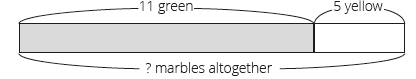2 (compare) Thelma has 14 apples. Ben has 4 more apples then Thelma. How many apples does Ben have?3 (change) Thelma had 4 mangos and her mother gave her 13 mangoes. How many mangoes does Thelma have now?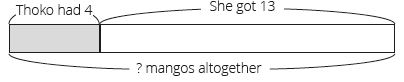Pupils attempt their class work STEP 4HOME-WORK Note: Learners solve this problem on their own at home. Teachers can draw bar diagramand go over the solution to the word problem in the next lesson.Solve the following addition problem: Martha has 10 dolls. Mary has 5 more dolls then Martha. How many dolls does Mary have?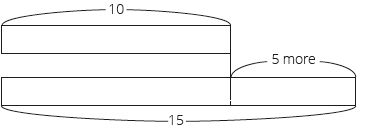The pupils writes it in their homework book STEP 5SUMMARY The teacher summarizes by reminding the pupils that in today’s lesson we solved word problems. We solved addition word problems of change, compare and combine. She marks their class works, makes corrections where necessary and commends them positively

PERIOD 5: Weekly Test/consolidations

TEACHER’S ACTIVITY: The teacher revises all the concepts treated from period 1-4 and gives the pupils follow through exercises, quiz and tests. She marks the exercises, makes corrections and commends the pupils positively.

PUPIL’S ACTIVITY: The pupils work on the worksheets and exercises give by the teacher individually

CONSOLIDATION

Calculate using the column method:

1 Complete the following:

65 – 32 = (60 + (5)) – (30 + (2))

= (60 – 30) + (5 – 2)

= 30 + 3

= ___

2 Calculate 65 – 44 = __ using the column method

3 Calculate 89 – 60 = ___ using the column method.

4 Show 67 – 17 = ___ using the number line.

5 Calculate 55 – 42 = __ using the column method.

6 Show 80 – 13 = ___ using the number line.